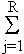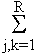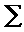Nonlinear Autoregressive Models and Fingerprints

## Nonlinear Autoregressive Models and Fingerprints

introduction
nonlinear modelling
NLAR fingerprints
significance testing
references
unsolved problems

### Introduction: Linear autoregressive models

In a linear autoregressive model of order R, a time series yn is modelled as a linear combination of R earlier values in the time series, with the addition of a correction term xn:
ynmodel = xn -aj yn-j .
The autoregressive coefficients aj (j = 1, ... R) are fit by minimizing the mean-squared difference between the modelled time series ynmodel and the observed time series yn. The minimization process results in a system of linear equations for the coefficients an, known as the Yule-Walker equations [Yule, G.U. (1927) On a method of investigating periodicities in disturbed series with special reference to Wolfer's sunspot numbers. Phil. Trans. Roy. Soc. Lond. A 226, 267-298].

### Nonlinear modelling

In a full nonlinear autoregressive model, quadratic (or higher-order) terms are added to the linear autoregressive model. A constant term is also added, to counteract any net offset due to the quadratic terms:
ynmodel = xn - a0 -aj yn-j -bj,k yn-jyn-k.
The autoregressive coefficients aj (j = 0, ... R) and bj,k (j, k = 1, ...., R) are fit by minimizing the mean-squared difference between the modelled time series ynmodel and the observed time series yn. The minimization process also results in a system of linear equations, which are generalizations of the Yule-Walker equations for the linear autoregressive model.

Conceptually, the time series yn is considered to be the output of a circuit with nonlinear feedback, driven by a noise xn. In principle, the coefficients bj,k describe dynamical features that are not evident in the power spectrum or related measures.

Although the equations for the autoregressive coefficients aj and bj,k are linear, the estimates of these parameters are often unstable, essentially because a large number of them must be estimated. This is the motivatation for the NLAR fingerprint.
introduction nonlinear modelling NLAR fingerprints significance testing references

### NLAR fingerprints

To create the nonlinear autoregressive fingerprint, only a single term of the full quadratic model is retained, along with the constant term:
ynmodel = xn - a0 -aj yn-j - bu,v yn-uyn-v.
The autoregressive coefficients aj (j = 0, ... R) and the single coefficient bu,v are fit by minimizing the mean-squared difference between the modelled time series ynmodel and the observed time series yn. This involves estimation of only R+2 parameters (compared with (R+1)(R+2)/2 equations for the full
quadratic autoregressive model), and substantially more reliable values for the parameters. However, like the full quadratic autoregressive model, it provides a characterization of the nonlinear dynamics of the time series.

The fitting procedure is performed sequentially for all pairs of lags u and v (u,v = 1, ...., R). The "NLAR fingerprint" consists (see example) of a contour map of the residuals|ynmodel - yn|2, parametric in the choice of lags u and v.
top introduction nonlinear modelling NLAR fingerprints significance testing references

### Significance testing

Introduction of an additional term into a linear or nonlinear autoregressive model always improves the fit (in the mean-squared sense). Akaike [Akaike, H. (1974) A new look at statistical model identification. IEEE Trans. Auto. Control AC-19,716-723] showed that, for a linear autoregressive model, a significant improvement in the fit is associated with a reduction in the residual variance of at least 2V/N, where V is the variance without the candidate additional term, and N is the number of data points.

We showed that the same criterion, a reduction in residual variance by at least 2V/N, is a criterion for the significance of a single nonlinear term as well.

### References

top introduction nonlinear modelling NLAR fingerprints significance testing